# Propositional Equivalences 1 Tautologies Contradictions and Contingencies A

• Slides: 12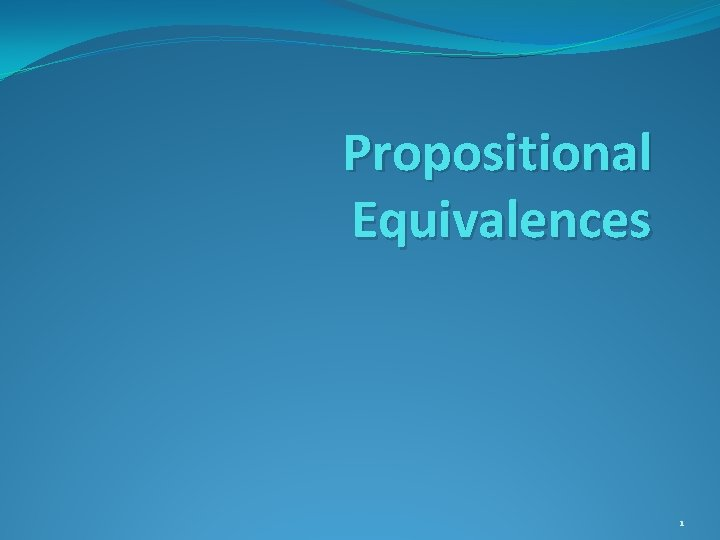Propositional Equivalences 1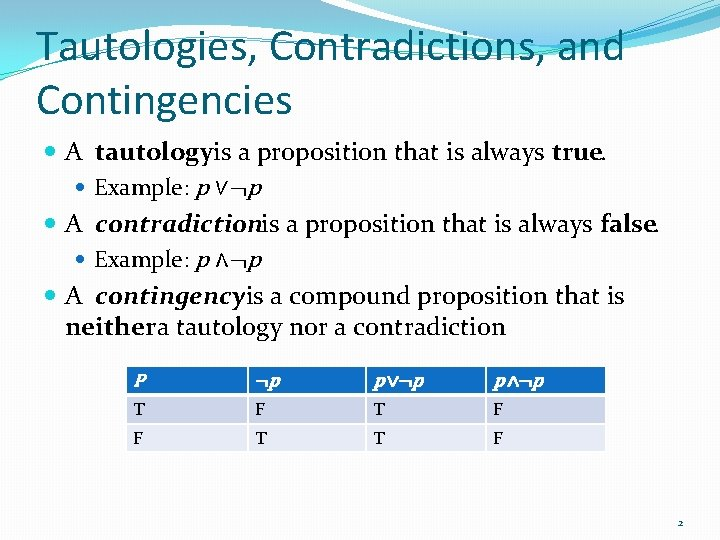Tautologies, Contradictions, and Contingencies A tautologyis a proposition that is always true. Example: p ∨¬p A contradictionis a proposition that is always false. Example: p ∧¬p A contingencyis a compound proposition that is neithera tautology nor a contradiction P ¬p p ∨¬p p ∧¬p T F F T T F 2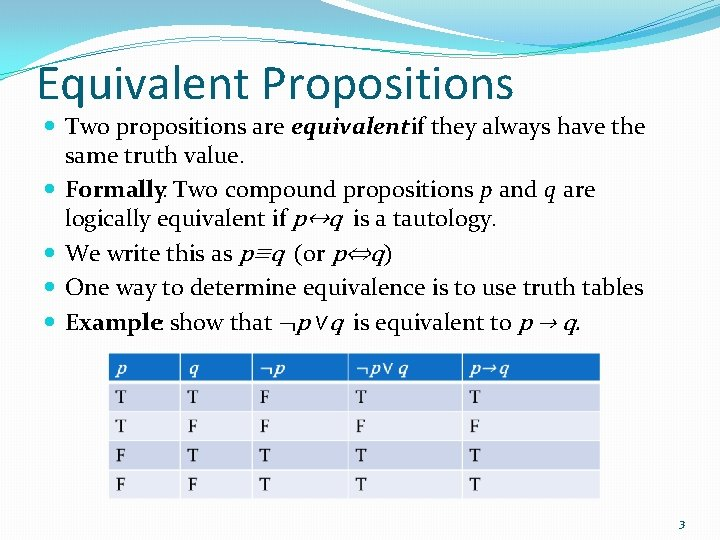Equivalent Propositions Two propositions are equivalentif they always have the same truth value. Formally: Two compound propositions p and q are logically equivalent if p↔q is a tautology. We write this as p≡q (or p⇔q) One way to determine equivalence is to use truth tables Example: show that ¬p ∨q is equivalent to p → q. 3Equivalent Propositions Example: Show using truth tables that implication is equivalent to its contrapositive Solution: 4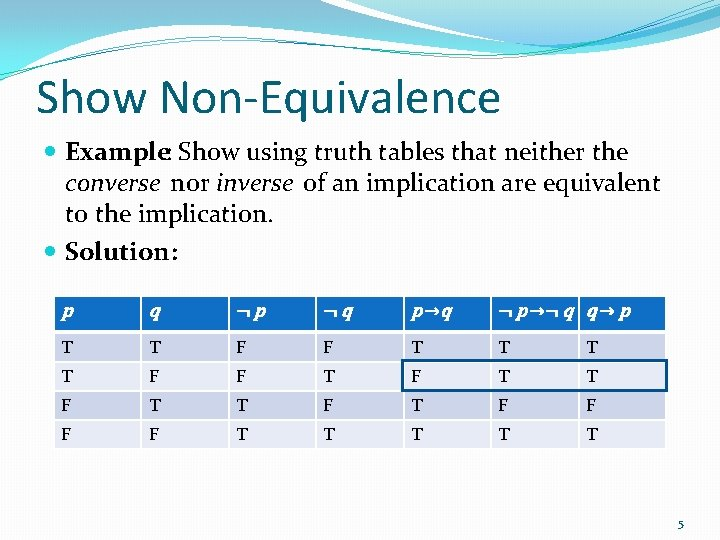Show Non-Equivalence Example: Show using truth tables that neither the converse nor inverse of an implication are equivalent to the implication. Solution: p q ¬p ¬q p →q ¬ p →¬ q q → p T T F F T T F T F F T T T 5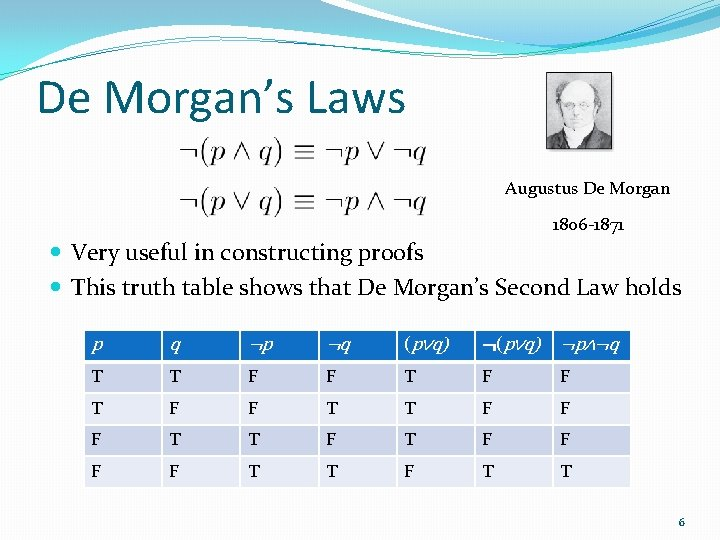De Morgan’s Laws Augustus De Morgan 1806 -1871 Very useful in constructing proofs This truth table shows that De Morgan’s Second Law holds p q ¬p ¬q (p∨q) ¬p∧¬q T T F F T T F T F F T T 6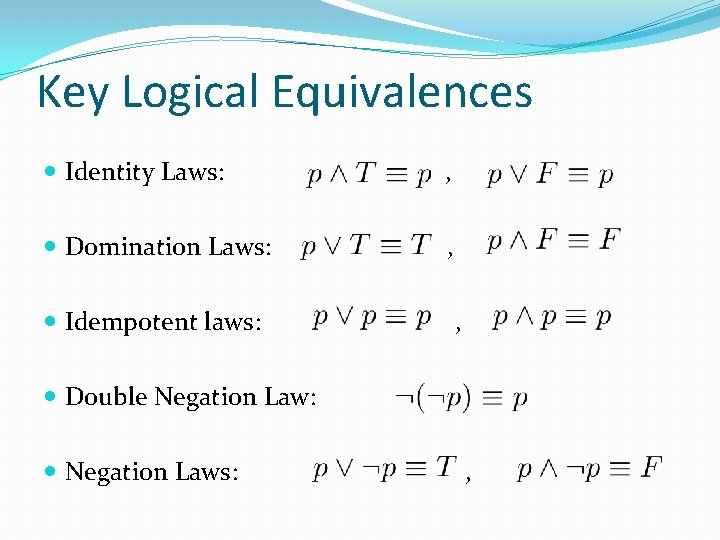Key Logical Equivalences Identity Laws: , Domination Laws: , Idempotent laws: , Double Negation Law: Negation Laws: ,Key Logical Equivalences (cont) Commutative Laws: Associative Laws: Distributive Laws: Absorption Laws: ,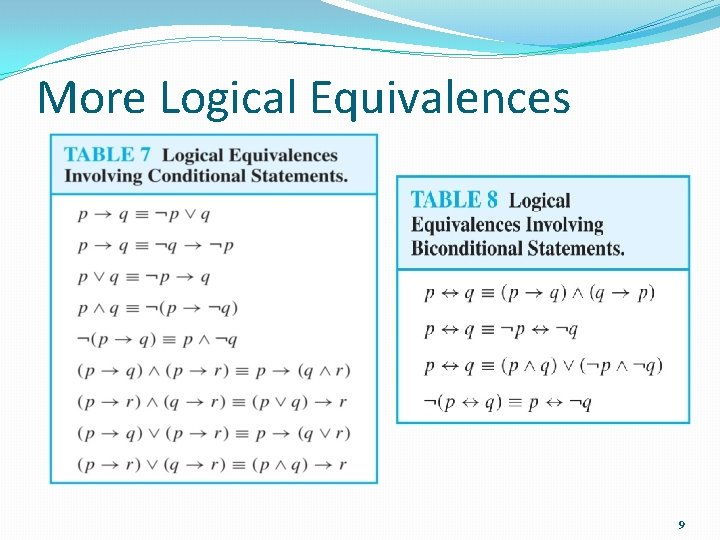More Logical Equivalences 9Equivalence Proofs Instead of using truth tables, we can show equivalence by developing a series of logically equivalent statements. To prove that A ≡B we produce a series of equivalences leading from A to B. Each step follows one of the established equivalences (laws) Each Ai can be an arbitrarily complex compound proposition. 10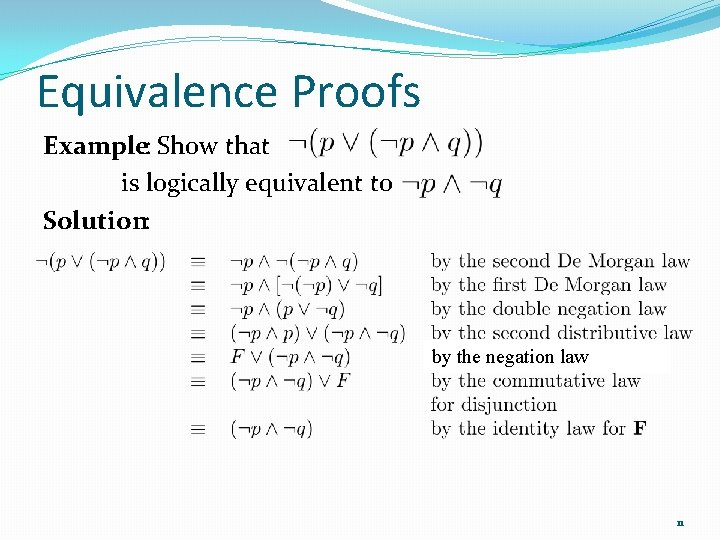Equivalence Proofs Example: Show that is logically equivalent to Solution: by the negation law 11Equivalence Proofs Example: Show that is a tautology. Solution: by equivalence from Table 7 (¬q ∨ q) by the negation law 12